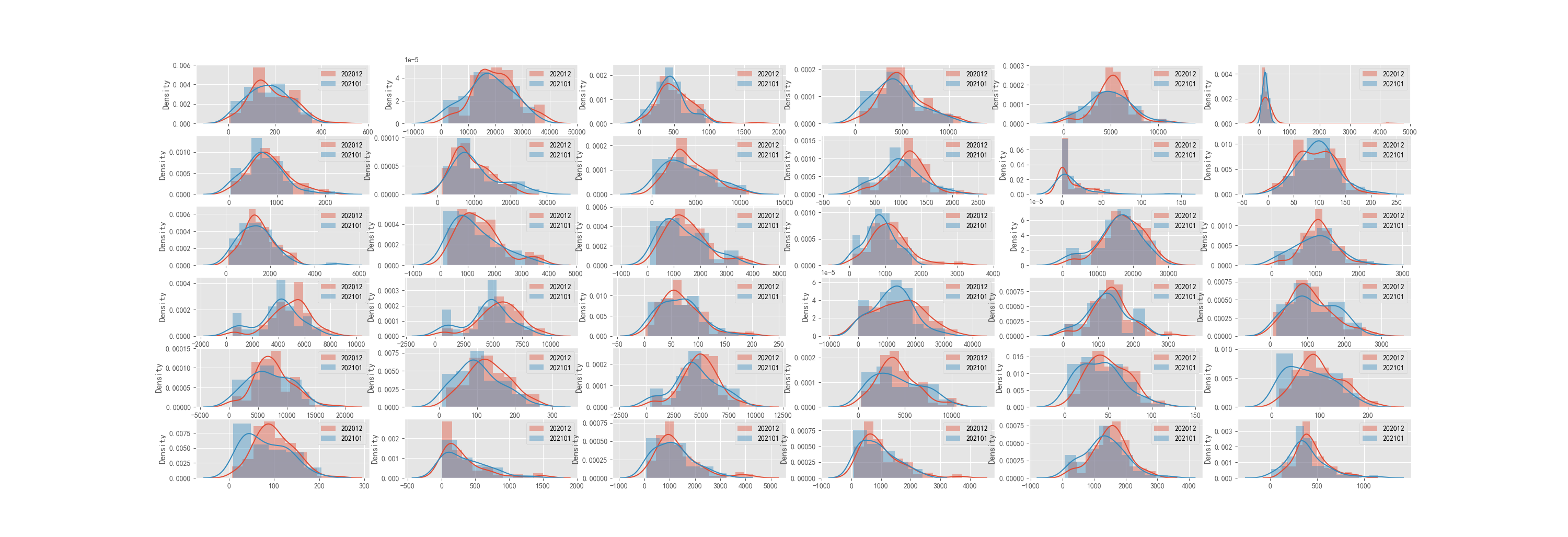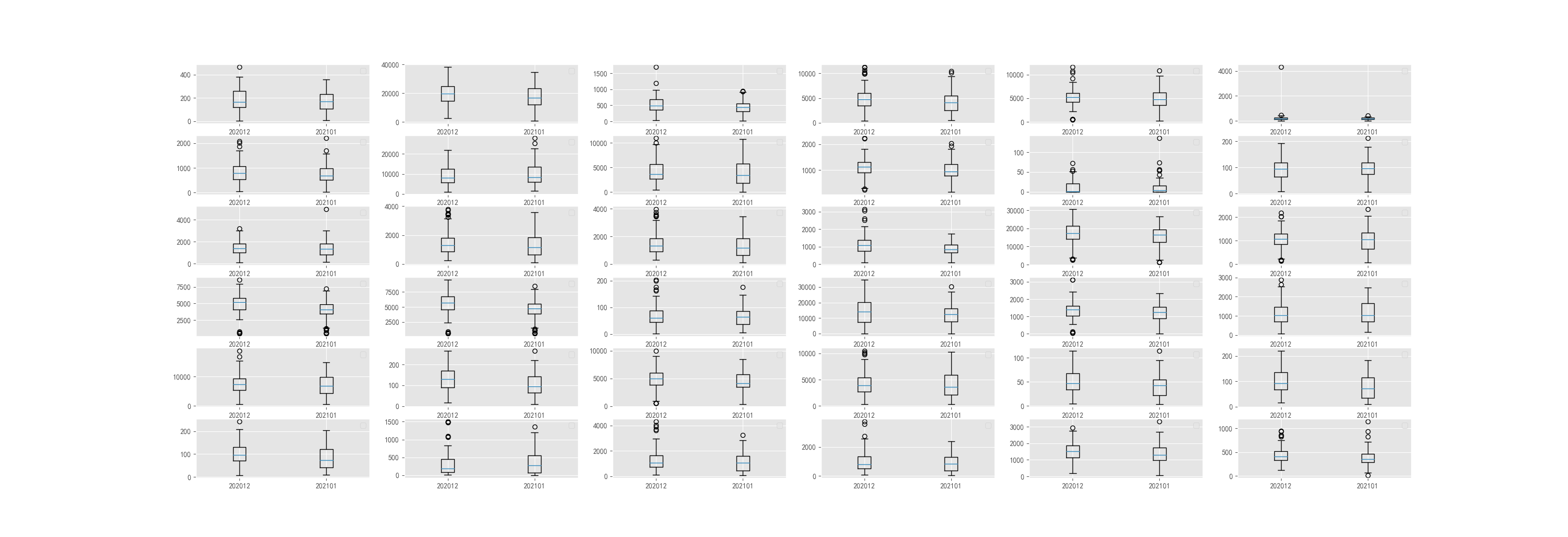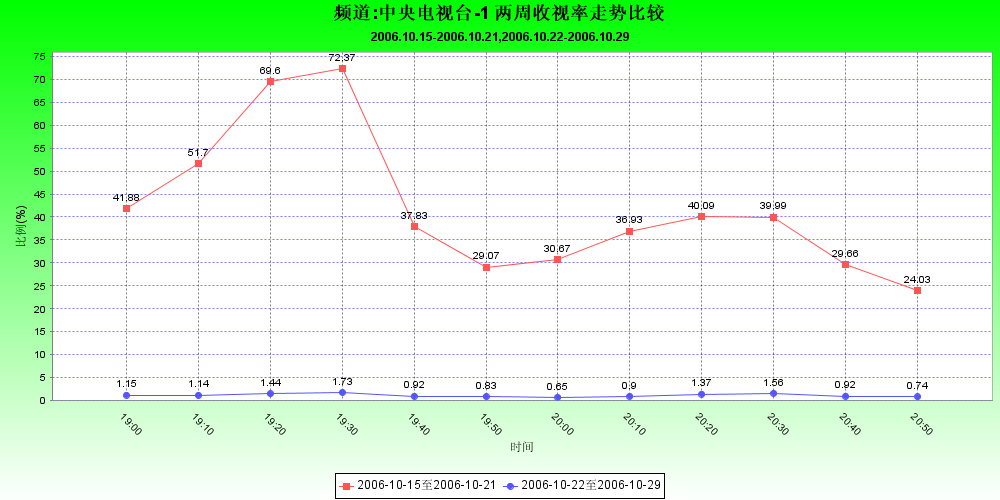• python画图 箱线图 累积图 密度曲线图 数据点 标签 图例图片代码 图片 话不多数，先上一波效果图 代码 # -*- coding: utf-8 -*- """ Created on Wed Jan 20 14:36:24 2021 @author: dujidan """ import numpy as...
python画图 箱线图 累积图 密度曲线图  数据点 标签 图例 合图图片代码
图片
话不多数，先上一波效果图代码
# -*- coding: utf-8 -*-
"""
Created on Fri Jan 22 08:33:24 2021

@author: dujidan
"""

import os
import numpy as np
import seaborn as sns
import matplotlib.pyplot as plt

plt.style.use("ggplot")
# 解决中文显示问题
plt.rcParams['font.sans-serif'] = ['SimHei']
plt.rcParams['axes.unicode_minus'] = False

def read_column_float_file(input_file, start_row=0, n_col='all'):
out_list = []
with open(input_file, encoding='utf-8') as f_in:
data_list = f_in.read().strip('\n').split('\n')
if n_col == 'all':
for line in data_list[start_row:]:
out_list.append([float(j) for j in line.split('\t') if j != ''])
else:
for line in data_list[start_row:]:
col_value = line.split('\t')[n_col]
if col_value != '':
out_list.append(float(col_value))
return out_list, data_list.split('\t')

# 箱线图，合图 2*2比较
def boxplot_2D_inOne(list_1, list_2, out_file='./boxplot_2D.png', suptitle_name='Box plot ', nrow=5, ncol=8):
"""
list_1, list_2: []  1D;  len(A) = len(B)
out_file: /home/test.png
"""
plt.figure(figsize=(50, 30))
for i in range(len(list_1)):
plt.subplot(nrow, ncol, i+1)
plt.boxplot((list_1[i], list_2[i]), labels=('before', 'after'))
plt.legend()
plt.suptitle(suptitle_name, fontsize=60)
plt.savefig(out_file, dpi=500)

# 密度曲线图，合图 2*2比较
def distplot_2D_inOne(list_1, list_2, out_file='./doxplot_2D.png', suptitle_name='Dist plot', nrow=5, ncol=8):
plt.figure(figsize=(50, 30))
for i in range(len(list_1)):
plt.subplot(nrow, ncol, i+1)
sns.distplot(list_1[i], kde=True, hist=True, label='before')
sns.distplot(list_2[i], kde=True, hist=True, label='after')
plt.legend()
plt.xlabel('深度')
plt.ylabel('密度曲线')
plt.suptitle(suptitle_name, fontsize=60)
plt.savefig(out_file, dpi=500)

def read_float_file(input_file, start_row=0):
out_list, index_list = [], []
with open(input_file, encoding='utf-8') as f_in:
data_list = f_in.read().strip().split('\n')
index_list = data_list
for line in data_list[start_row:]:
out_list.append([float(j) for j in line.split('\t') if j != ''])
return out_list, index_list

def plot_percent_line(all_list, index_list):
"""
all_list：2D list
index_list：图例名称列表
"""
fig, ax = plt.subplots()
label_count = -1
x_ticks = np.arange(0, 1, 0.1)
for a_list in all_list:
label_count += 1
ret_list = []
for set_num in x_ticks:
count = 0
for i in a_list:
if i >= set_num:
count += 1
ret_list.append(count/len(a_list))

ax.plot(x_ticks, ret_list, 'o-', label=index_list[label_count])
plt.xticks(x_ticks)
for i, txt in enumerate(ret_list):
ax.annotate(txt, (x_ticks[i], ret_list[i]))
print(x_ticks[i], ret_list[i])
plt.legend()
plt.title('score 累计趋势')
plt.xlabel(' 得分')
plt.ylabel('> 得分 的位点占比')
plt.savefig('得分统计分布-累计图.png', dpi=500)

if __name__ == "__main__":
depth_file_20 = '202012_all_depth.xls'
depth_file_21 = '202101_all_depth.xls'
list_1, __ = read_column_float_file(depth_file_20, start_row=1, n_col='all')
list_2, __ = read_column_float_file(depth_file_21, start_row=1, n_col='all')
distplot_2D_inOne(list_1, list_2, out_file='./doxplot_2D.png', suptitle_name='Dist plot', nrow=5, ncol=8)
boxplot_2D_inOne(list_1, list_2, out_file='./boxplot_2D.png', suptitle_name='Box plot ', nrow=5, ncol=8)

# score
score_file = 'score.xls'
a_list, __ = read_column_float_file(score_file, start_row=1, n_col=0)
b_list, __ = read_column_float_file(score_file, start_row=1, n_col=1)
c_list, __ = read_column_float_file(score_file, start_row=1, n_col=2)
d_list, index_list = read_column_float_file(score_file, start_row=1, n_col=3)

plt.boxplot((a_list, b_list, c_list, d_list), labels=(index_list))
plt.title('得分统计分布-箱线图')
plt.savefig('得分统计分布-箱线图.png', dpi=500)

plt.title('得分统计分布-密度图')
sns.distplot(a_list, label=index_list)
sns.distplot(b_list, label=index_list)
sns.distplot(c_list, label=index_list)
sns.distplot(d_list, label=index_list)
plt.legend()
plt.savefig('得分统计分布-密度图.png', dpi=500)

all_list = [a_list, b_list, c_list, d_list]
plot_percent_line(all_list, index_list




展开全文• 在工作中用到了生成曲线图时要将数据值显示在每个数据点上，并且不同数据线的数据点要显示不同的小图标，经过长时间的查找资料研究终于实现了功能。工作中就生成了如下的图片：生成此图中的数据点图标和显示数据值的...
在工作中用到了生成曲线图时要将数据值显示在每个数据点上，并且不同数据线的数据点要显示不同的小图标，经过长时间的查找资料研究终于实现了功能。工作中就生成了如下的图片：生成此图中的数据点图标和显示数据值的主要代码就是下面这些，这里使用的是DefaultCategoryDataset数据集：

CategoryPlot plot=chart.getCategoryPlot();                 plot.setBackgroundPaint(Color.WHITE);                plot.setRangeGridlinePaint(Color.BLUE);//纵坐标格线颜色                plot.setDomainGridlinePaint(Color.BLACK);//横坐标格线颜色                plot.setDomainGridlinesVisible(true);//显示横坐标格线                plot.setRangeGridlinesVisible(true);//显示纵坐标格线                                                            LineAndShapeRenderer renderer = (LineAndShapeRenderer)plot.getRenderer();                DecimalFormat decimalformat1 = new DecimalFormat("##.##");//数据点显示数据值的格式                renderer.setItemLabelGenerator(new StandardCategoryItemLabelGenerator("{2}", decimalformat1));                                                  //上面这句是设置数据项标签的生成器                renderer.setItemLabelsVisible(true);//设置项标签显示                renderer.setBaseItemLabelsVisible(true);//基本项标签显示                         //上面这几句就决定了数据点按照设定的格式显示数据值                  renderer.setShapesFilled(Boolean.TRUE);//在数据点显示实心的小图标                renderer.setShapesVisible(true);//设置显示小图标

有用到的网友可以试一下。
展开全文jfreechart
• 在工作中用到了生成曲线图时要将数据值显示在每个数据点上，并且不同数据线的数据点要显示不同的小图标，经过长时间的查找资料研究终于实现了功能。工作中就生成了如下的图片：  生成此图中的数据点图标和显示...
      在工作中用到了生成曲线图时要将数据值显示在每个数据点上，并且不同数据线的数据点要显示不同的小图标，经过长时间的查找资料研究终于实现了功能。工作中就生成了如下的图片：生成此图中的数据点图标和显示数据值的主要代码就是下面这些，这里使用的是DefaultCategoryDataset数据集：
CategoryPlot plot=chart.getCategoryPlot();
plot.setBackgroundPaint(Color.WHITE);
plot.setRangeGridlinePaint(Color.BLUE);//纵坐标格线颜色
plot.setDomainGridlinePaint(Color.BLACK);//横坐标格线颜色
plot.setDomainGridlinesVisible(true);//显示横坐标格线
plot.setRangeGridlinesVisible(true);//显示纵坐标格线

LineAndShapeRenderer renderer = (LineAndShapeRenderer)plot.getRenderer();
DecimalFormat decimalformat1 = new DecimalFormat("##.##");//数据点显示数据值的格式
renderer.setItemLabelGenerator(new StandardCategoryItemLabelGenerator("{2}", decimalformat1));
//上面这句是设置数据项标签的生成器
renderer.setItemLabelsVisible(true);//设置项标签显示
renderer.setBaseItemLabelsVisible(true);//基本项标签显示
//上面这几句就决定了数据点按照设定的格式显示数据值
renderer.setShapesFilled(Boolean.TRUE);//在数据点显示实心的小图标
renderer.setShapesVisible(true);//设置显示小图标


展开全文jfreechart plot
• 一开始取值并显示数据的时候，还算流畅，但是当过了三四十秒后，四条曲线上面的多了，显示的时候就开始卡顿了，有什么办法能够让这个实时曲线图数据采集频率一致，并且不卡顿界面的办法？ ![图片说明]...asp.net c# microsoft
• 生成此中的数据点图标和显示数据值的主要代码就是下面这些，这里使用的是DefaultCategoryDataset数据集：    CategoryPlot plot=chart.getCategoryPlot();生成此图中的数据点图标和显示数据值的主要代码就是下面这些，这里使用的是DefaultCategoryDataset数据集：

CategoryPlot plot=chart.getCategoryPlot();
plot.setBackgroundPaint(Color.WHITE);
plot.setRangeGridlinePaint(Color.BLUE);//纵坐标格线颜色                plot.setDomainGridlinePaint(Color.BLACK);//横坐标格线颜色                plot.setDomainGridlinesVisible(true);//显示横坐标格线                plot.setRangeGridlinesVisible(true);//显示纵坐标格线

LineAndShapeRenderer renderer = (LineAndShapeRenderer)plot.getRenderer();
DecimalFormat decimalformat1 = new DecimalFormat("##.##");//数据点显示数据值的格式                renderer.setItemLabelGenerator(new StandardCategoryItemLabelGenerator("{2}", decimalformat1));
//上面这句是设置数据项标签的生成器                renderer.setItemLabelsVisible(true);//设置项标签显示                renderer.setBaseItemLabelsVisible(true);//基本项标签显示
//上面这几句就决定了数据点按照设定的格式显示数据值                  renderer.setShapesFilled(Boolean.TRUE);//在数据点显示实心的小图标                renderer.setShapesVisible(true);//设置显示小图标

展开全文jfreechart
• 本软件是绿色软件，可以直接安装。主要功能是能将图形的曲线选中的，快速转换成你需要的数据
• jfreechart+sql实现时间曲线图(显示曲线数据点)收藏备忘一、JFreeChart获取。 JFreeChart是JFreeChart公司在开源网站SourceForge.net上的一个项目，该公司的主要产品有如下： 1、JFreeReport：报表解决工具 2、...jfreechart sql string import sqlserver
• 解决曲线图或者折线图在两端中间没有数据时无法绘画成线的问题源码修改， 解决方案： 在数据之间填充假数据，并且创建一个和数据同级的 list 来验证是不是假数据，如果是假数据就不绘制，是真数据才绘制，...
• 近日由于项目需要，学习了DynamicDataDisplay实现动态曲线图，网上的资料基本上够用了，就是双击获得数据点没能找到资料，只好下载了DynamicDataDisplay的源码来学习。总结共享如下： 1、xaml定义wpf object 项目管理 timer
• Curve.exe程序用于曲线形式查看工程试验中生成的文本文件数据， 程序在界面中设定了各种曲线变换操作和数据处理功能，便于分析; 算法对前级曲线数据进行处理,生成新数据以本级曲线形式显示; 原数复制: 不做任何处理...
• 今天写机械设计的带传动实验报告时，需要绘制滑动曲线ε-F和效率曲线η-F的关系曲线——正在学MATLAB的我内心狂喜，直接复制表格中的数据，掏出了plot函数。>> S=[0.348.55.50.364.64.80.380.630.780.63.34....matlab排序
• 首先通过自己在文献或者百度上搜索到的图片，得到大体的数据点，在把数据点扩展扩展，比如大概得到50个点，那么我想要500个点怎么办？把每个点都十等分，线性求值。 由曲线得到数据，我使用了一款软件，叫做...
• 本程序为基于MATLAB GUI 创建的三维数组插值程序。含有数据点，三维图形，可选择多种插值方法:linear, nearest, spline, cubic。
• ![图片说明](https://img-ask.csdn.net/upload/201907/26/1564111306_761928.jpg) 比如根据这个图片里的曲线，自动生成数据点？ 以前没做过python，导师给安排的任务一头雾水，求各位大佬指点方向。python
• 上谷歌查了查，对于曲线图提取数据基本上是手动在曲线上取几个，然后由这个几个开始遍历领域，判断领域是否是黑色，然后再遍历这个黑色的领域，最终找出一条最长的线便是曲线，一种类似曲线拟合的做法？...
• 本程序可对论文/产品手册等资料中的各类...使用时，首先对图片坐标轴四进行定位，随后按照提示进行操作即可完成数据提取。 "I thought what I'd do was I'd pretend I was one of those deaf-mutes, or should I?
• Matlab手动取图形曲线数据并重画 http://www.docin.com/p-1129028185.html
• /** **QQ:252574345 **MSN:lele_love_lomboz@hotmail.com */        XYPlot xyplot=(XYPlot) chart.getPlot();  //设置数据点可见  xylinerenderer.setShapesVisible(true); xy...jfreechart Java
• 详细介绍使用MFC读取txt数据画曲线图方法,并列举例程代码供参考.关于MFC读取txt数据，并且画曲线图记事本中保存的...现在想把数据读出来，并且画曲线图到已经创建好的对话框上，希望画出坐标轴和数据点曲线，要这么做
• 利用第三方的AChartEngine来进画曲线图数据在链表ArrayList中，想问的是怎么实现每在list中取一个数，就能在曲线图中更新相应的呢？
• web曲线图,可以查看某数据,可以拖曳时间轴,简单易学
• 在IOS项目中，用到corePlot这个第三方库，曲线图应经成型，但是不知道怎么在的位置添加所对应的数据。。。请各位大神帮帮忙。。谢了coreplot
• 原文地址：Matlab手动取图形曲线数据并重画作者：ronei一次，在一篇paper上看到一张，可惜里面的曲线数据没有提供。 那么如何利用这张图，自己获取数据呢？ 比如有下面这么一张，我们要用鼠标取得曲线的数值，...matlab 可视化 oracle 数学建模 webgl
• 　为了操作和应付变化，所以将绘制曲线图的功能单独封装成一个类，里面的数据完全是模拟的，在横向坐标上每个像素间隔用一个来控制（实际中可能会加大这个距离），横向是个随机生成的数（实际开发中这应该来自我们...
• 写论文时，经常需要用Origin或者Matlab把实验数据画成曲线并导出保存。然而，只得到实验数据还没完，有时还需要和...手动提取数据点吗？太花时间了！关键很烧眼睛。今天给大家推荐这样一款软件，可以实现从图片上的...
• 在上一篇博客中，我们首先讨论了论文以及产品手册中常见的曲线图的大致分类，并分析了每类图片的特点。以此为基准，我们将这些图片分为两类处理。对于第一类图片，由于曲线变化较为简单，在曲线上所需提取的数据点...图像处理 MATLAB...